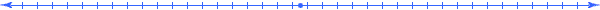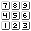###### How to Solve Word Problems Programmatically

Chapter

11Chapter 11.  Proportional Models

 11.1 Direct Division 11.2 Rate Example 11.3 Percent Example 11.4 Price Example 11.5 Probability Example 11.6 Ratio Example 11.7 Fraction Example 11.8 Linked Definition Example 11.9 Activities and Explorations

11.1

#### Direct Divisionrates percents prices probabilities ratios fractions of proportions Keywords that Indicate Proportionality

Most problems in real life involve simple proportional relationships.  In fact, you may find it surprising how easy it is to handle rates, percents, prices, probabilities, ratios, “fractions of”, and proportions when we use Unified Math®.  In proportional relationships, the expression in the Relationship column results in the same division as in the meaning of the constant of proportionality.  Keep in mind that the even the physical world around us is highly proportional as illustrated by the Newton Laws of Motion, Ideal Gas Laws, Weights on a Balance, etc.

11.2

#### Rate ExampleA car travels 50 miles per hour.  How long does it take to go 150 miles? Word problem involving a rate

 Qty Value Meaning Relationship q1 x hr q2 50 mi/hr q3 150 mi

In the above table, we identify (Step 1) the quantities (x, 50, and 150) and measure (Step 2) them by assigning meaning expressions.

 Qty Value Meaning Relationship q1 x hr q2 50 mi/hr q3 / q1 q3 150 mi

Formulate:  50 = 150/x

Solve:

50*x = 150

x = 3

We relate (Step 3) the quantities by observing that the meaning “mi/hr” in the first row involves proportionality and we divide the “mi” in the meaning of quantity q3  by the “hr” in the meaning of quantity q1.

We formulate (Step 4) an equation from the first line in the table by equating the expression in the Quantity column with the expression in the Relationship column:

50 = 150 / x          or rearranged as         50 x = 150

We then solve (Step 5) this equation for the variable, x = 3.

11.3

#### Percent ExampleIf we have 40 dimes, what percent of 200 coins would the dimes be? Word problem involving a percent

 Qty Value Meaning Relationship q1 x /100 dimes/coins q2/q3 q2 40 dimes q3 200 coins

Formulate:  x/100 = 40/200

Solve:  x = 80

The problem asks for “what percent” so the variable x combines with 100 in the value column to create a “x per 100” or in other words “x percent”.    This results in the formula:  x/100 = 40/200  where we solve and get x = 20.

11.4

#### Price ExampleIf Jane had \$50.00 to spend on apples, how much can she purchase at a price of \$ 0.25 per pound? Word problem involving a price

 Qty Value Meaning Relationship q1 x lb q2 50 dol q3 0.25 dol/lb q2 / q1

Formulate:  0.25 = 50 / x

Solve:

0.25 * x = 50

x = 200

11.5

#### Probability ExampleIf 300 out of 1000 people polled preferred the new product, what is the probability of that the next person polled will prefer the new product? Word problem involving a probability

 Qty Value Meaning Relationship q1 p people who prefer/people polled q2/q3 q2 300 people who prefer q3 1000 people polled

Formulate:  p = 300 / 1000

Solve:  x = .3

11.6

#### Ratio ExampleThe salad dressing recipe mixes vinegar with oil in a ratio of 2 to 5 (that is 2 parts of vinegar to 5 parts of oil).   How much vinegar do we need to mix with 20 fluid ounces of oil? Word problem involving a ratio

 Qty Value Meaning Relationship q1 x [oz~Volume(Vinegar)] q2 2/5 [oz~Volume(Vinegar)]/[oz~Volume(Oil)] q1/q3 q3 20 [oz~Volume(Oil)]

The ration of 2 to 5 parts (sometimes written as 2:5) in this problem indicates a proportional relationship between the amount of vinegar to the amount of oil.  The constant of proportionality comes from dividing the numbers in the ratio.  When we construct the meaning of the constant of proportionality we must put the meaning of the numerator number in the numerator of the meaning, and similarly for the denominator.  On constructing the expression in the relationship column, we again make sure that the meanings line up (the x in the numerator corresponds to its meaning  [oz~Volume(Vinegar)] in the numerator and the 20 in the denominator corresponds to its meaning [oz~Volume(Oil)] in the denominator.

Formulate:  2/5 = x / 20

Solve:  x = (2/5)*20 = 8

11.7

#### Fraction ExampleThe fraction of people purchasing the maintenance contract has increased to 2/7 during the last month.  If the company sold 140 appliances during the last month, how many maintenance contracts did they sell? Word problem involving a fraction

 Qty Value Meaning Relationship q1 x contracts q2 2/7 contracts/appliances q1/q3 q3 140 appliances

The fraction 2/7 indicates a proportional relationship between the instances of contracts and the instances of appliances.  This fraction determines the constant of proportionality with a meaning having the numerator 2 associated with "contracts" and the 7 associated with "appliances".  On constructing the expression in the relationship column, we again make sure that the meanings line up with the x in the numerator corresponds to its meaning "contracts" in the numerator and the 140 in the denominator corresponds to its meaning "appliances" in the denominator.

Formulate:  2/7 = x / 140

Solve:  x = (2/7)*140 = 40

11.8

#### Linked Definition ExampleThe odometer of a new automobile functions improperly and registers only 2 miles for every 3 miles driven.  If the odometer increases 48 miles, how many miles has the automobile actually been driven? Word problem involving a fraction

 Qty Value Meaning Relationship q1 x [mi~Driven] q2 2/3 [mi~Odometer]/[mi~Driven] q3 / q1 q3 48 [mi~Odometer]

Formulate:  2/3  = 48 /  x

Solve:

2/3 * x  = 48

x = 72

The meaning of 2 is not just "miles", but it's definition involves the 3.  Similarly, the meaning of 3 depends on 2.  The words "for every" mean the same as "per" so this reduces to a rate-type of problem.

The characters depicted in red indicate some abbreviations that would still completely determine the model.  The user can use any abbreviations they desire as long as they remain consistent and avoid ambiguity.

11.9

#### Activities and ExplorationsActivities:

 Read through the explanations of various ratio problems http://mathforum.org/library/drmath/sets/select/dm_mixture.html Notice his use of units analysis and the domino approach in the problem: http://mathforum.org/library/drmath/view/58011.html   Solve Proportional Word Problems Use the meaning table to solve various problems that involve different types of proportionality using websites and resources like: http://www.swt.edu/slac/math/wordprb/wordprb.html http://www.beavenandassociates.com/pdfs/Review%20of%20Word%20Problems1.pdf http://www.accd.edu/sac/math/FACULTY/ELennon/M0301f/ReadG_J.doc   Preparing For Exams http://www.all-mba.com/gre-guidec7view1d.html (Printable version at:  http://www.all-mba.com/gre-guidec7.html) Use the meaning table to solve the problems discussed in the context of taking multiple-choice tests.  Compare not only your answers but the process you went through to get the answer.  See also: http://www.novapress.net/diagnostic/gre_test/word.html

Explorations:

 Review Rate Word Problems http://www.garlikov.com/math/RateTime.html http://www.swt.edu/slac/math/wordprb/wordprb.html

Exercises:

 Do Related Problems In Your Textbook   Solve constant-rate word problems. Solve the following Proportional Word Problems

Copyright © 2004  Dr. Ranel E. Erickson